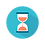From: Bayesian Models for Astrophysical Data, Cambridge Univ. Press

(c) 2017,  Joseph M. Hilbe, Rafael S. de Souza and Emille E. O. Ishida

you are kindly asked to include the complete citation if you used this material in a publication

Code 10.11 Beta model in Python using Stan, for accessing the relationship between the fraction of atomic gas and the galaxy stellar mass

==================================================================================

import numpy as np
import pandas as pd
import pystan
import statsmodels.api as sm

# built atomic gas fraction
y = np.array([data_frame['M_HI'][i] / (data_frame['M_HI'][i] + data_frame['M_STAR'][i])
for i in range(data_frame['M_STAR'].shape)])

x = np.array([np.log(item) for item in data_frame['M_STAR']])

# prepare data for Stan
data = {}
data['Y'] = y
data['nobs'] = data['X'].shape
data['K'] = data['X'].shape

# Fit
stan_code="""
data{
int<lower=0> nobs;                                         # number of data points
int<lower=0> K;                                              # number of coefficients
matrix[nobs, K] X;                                           # stellar mass
real<lower=0, upper=1> Y[nobs];                   # atomic gas fraction
}
parameters{
vector[K] beta;                                                 # linear predictor coefficients
real<lower=0> theta;
}
model{
vector[nobs] pi;
real a[nobs];
real b[nobs];

for (i in 1:nobs){
pi[i] = inv_logit(X[i] * beta);
a[i]  = theta * pi[i];
b[i]  = theta * (1 - pi[i]);
}

# priors and likelihood
for (i in 1:K) beta[i] ~ normal(0, 100);
theta ~ gamma(0.01, 0.01);

Y ~ beta(a, b);
}
"""

# Run mcmc
fit = pystan.stan(model_code=stan_code, data=data, iter=7500, chains=3,
warmup=5000, thin=1, n_jobs=3)

# Output
print(fit)

==================================================================================Output on screen:

3 chains, each with iter=7500; warmup=5000; thin=1;
post-warmup draws per chain=2500, total post-warmup draws=7500.

mean     se_mean         sd        2.5%        25%         50%        75%      97.5%      n_eff       Rhat
beta        9.24         4.0e-3      0.17          8.9         9.12          9.24        9.36         9.57       1859         1.0
beta       -0.42        1.8e-4    7.7e-3      -0.44        -0.43         -0.42      -0.42        -0.41       1856         1.0
theta          11.68        7.8e-3       0.37     10.96        11.43        11.67      11.92       12.43        2308        1.0
lp__         1165.4           0.03      1.17    1162.4      1164.9      1165.7    1166.3     1166.7        1822        1.0

Samples were drawn using NUTS at Wed May  3 18:56:51 2017.
For each parameter, n_eff is a crude measure of effective sample size,
and Rhat is the potential scale reduction factor on split chains (at
convergence, Rhat=1).